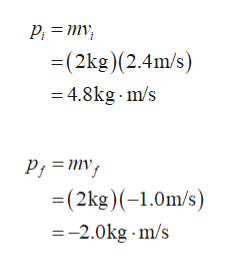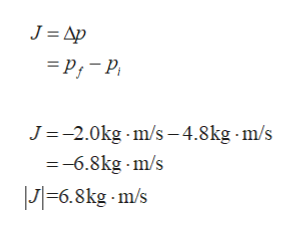# A 2 kg ball is moving at 2.4 m/s perpendicular to a wall. Upon hitting the wall, the ball rebounds at 1.0 m/s in the opposite direction. What is the magnitude of the impulse of the ball? (units in kg m/s)

Question
1 views

A 2 kg ball is moving at 2.4 m/s perpendicular to a wall. Upon hitting the wall, the ball rebounds at 1.0 m/s in the opposite direction. What is the magnitude of the impulse of the ball? (units in kg m/s)

check_circle

star
star
star
star
star
1 Rating
Step 1

The initial momentum of the ball is.

The final velocity of the ball rebounds in opposite direction so the final momentum is,help_outlineImage TranscriptioncloseP; = mv; =(2kg)(2.4m/s) = 4.8kg - m/s P; = mv; =(2kg)(-1.0m/s) =-2.0kg m/s fullscreen
Step 2

The impulse imparted to the ball is,

Substitute the values, to fi...help_outlineImage TranscriptioncloseJ = Ap = P;- P, J=-2.0kg - m/s – 4.8kg m/s =-6.8kg - m/s |J|=6.8kg - m/s fullscreen

### Want to see the full answer?

See Solution

#### Want to see this answer and more?

Solutions are written by subject experts who are available 24/7. Questions are typically answered within 1 hour.*

See Solution
*Response times may vary by subject and question.
Tagged in

### Newtons Laws of Motion